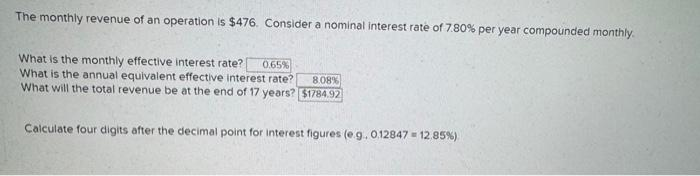Home / Expert Answers / Civil Engineering / the-monthly-revenue-of-an-operation-is-476-consider-a-nominal-interest-rate-of-7-80-per-year-comp-pa163

# (Solved): The monthly revenue of an operation is $476. Consider a nominal interest rate of 7.80% per year comp ... The monthly revenue of an operation is$476. Consider a nominal interest rate of 7.80% per year compounded monthly. What is the monthly effective interest rate?

What is the annual equivalent effective interest rate?

What will the total revenue be at the end of 17 years?

Calculate four digits after the decimal point for interest figures (e.g., 0.12847 = 12.85%).The monthly revenue of an operation is . Consider a nominal interest rate of per year compounded monthly. What is the monthly effective interest rate? What is the annual equivalent effective interest rate? What will the total revenue be at the end of 17 years? Calculate four digits after the decimal point for interest figures

We have an Answer from Expert